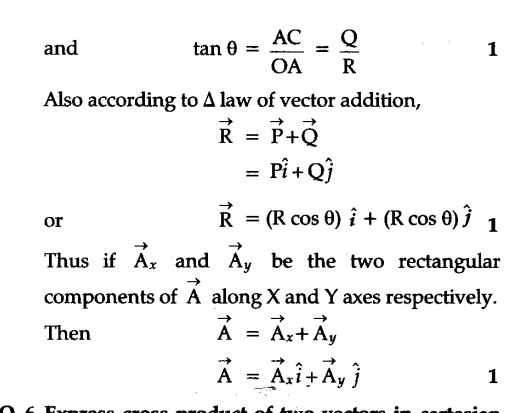# What do you understand by rectangular resolution of a vector ? Resolve it into its two rectangular components

It is defined as the factors of splitting a given vector in two or three component vectors at right angles to each other. The component vectors are called rectangular component of the given vector. Let R be the given vector acting in X-Y plane at an angle